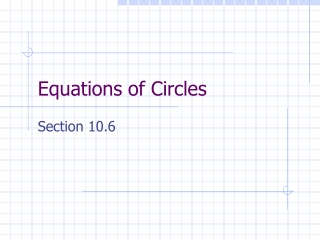Download PresentationEquations of Circles

# Equations of Circles - PowerPoint PPT PresentationDownload Presentation## Equations of Circles

- - - - - - - - - - - - - - - - - - - - - - - - - - - E N D - - - - - - - - - - - - - - - - - - - - - - - - - - -
##### Presentation Transcript

1. Equations of Circles Section 10.6

2. Essential Questions • How do I write the equation of a circle? • How do I use the equation of a circle and its graph to solve problems?

3. Find the length of r, the radius, in terms of the endpoints:

4. Standard Equation of a Circle ris the radius of the circle, (h, k)is the center of the circle, (x, y)is any point on the circle. Standard equation of a circle:

5. Example 1: Write the standard equation of the circle with a center (-4, 0) and radius 7.1.

6. Problem 1 • Write the standard equation of a circle with the given center and radius: center (-1, -3), and radius 6

7. Try This! • Give the center and radius of the circle: Center:(-5, -3) Radius:1

8. Example 2 - Give the Center & Radius of the Circle

9. Try this! Give the Center & Radius of the Circle:

10. Let's Investigate These Graphs!

11. Try this! • Use the information to write the standard equation of a circle: The center is (0, 0) , a point on the circle is (0, 4). The center is (-3, 6) and the diameter is 10.

12. Try This! • The equation of a circle is Tell whether each point is on the circle, in the interior of the circle, or in the exterior of the circle. 1. (0, 0) exterior 2. (3, -4) interior 3. (3, 1) on

13. Homework!!